Download Presentation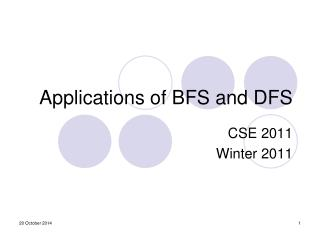Applications of BFS and DFS

# Applications of BFS and DFS - PowerPoint PPT Presentation

Applications of BFS and DFS. CSE 2011 Winter 2011. Some Applications of BFS and DFS. BFS To find the shortest path from a vertex s to a vertex v in an unweighted graph To find the length of such a path To find out if a strongly connected directed graph contains cyclesI am the owner, or an agent authorized to act on behalf of the owner, of the copyrighted work described.
Download Presentation## Applications of BFS and DFS

An Image/Link below is provided (as is) to download presentation

Download Policy: Content on the Website is provided to you AS IS for your information and personal use and may not be sold / licensed / shared on other websites without getting consent from its author.While downloading, if for some reason you are not able to download a presentation, the publisher may have deleted the file from their server.

- - - - - - - - - - - - - - - - - - - - - - - - - - E N D - - - - - - - - - - - - - - - - - - - - - - - - - -
Presentation Transcript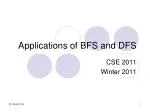### Applications of BFS and DFS

CSE 2011

Winter 2011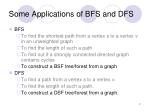Some Applications of BFS and DFS
• BFS
• To find the shortest path from a vertex s to a vertex v in an unweighted graph
• To find the length of such a path
• To find out if a strongly connected directed graph contains cycles
• To construct a BSF tree/forest from a graph
• DFS
• To find a path from a vertex s to a vertex v.
• To find the length of such a path.
• To construct a DSF tree/forest from a graph.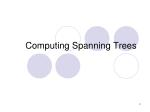### Computing Spanning Trees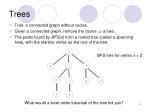Trees
• Tree: a connected graph without cycles.
• Given a connected graph, remove the cycles  a tree.
• The paths found by BFS(s) form a rooted tree (called a spanning tree), with the starting vertex as the root of the tree.

BFS tree for vertex s = 2

What would a level-order traversal of the tree tell you?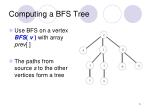Computing a BFS Tree
• Use BFS on a vertex BFS( v ) with array prev[ ]
• The paths from source s to the other vertices form a tree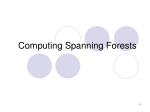### Computing Spanning Forests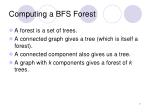Computing a BFS Forest
• A forest is a set of trees.
• A connected graph gives a tree (which is itself a forest).
• A connected component also gives us a tree.
• A graph with k components gives a forest of k trees.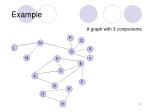Example

A graph with 3 components

P

Q

N

L

R

O

M

s

D

E

C

A

F

B

K

G

H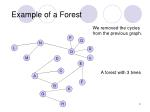Example of a Forest

We removed the cycles from the previous graph.

P

Q

N

L

R

O

M

s

D

E

C

A forest with 3 trees

A

F

B

K

G

H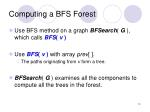Computing a BFS Forest
• Use BFS method on a graph BFSearch( G ), which calls BFS( v )
• Use BFS( v ) with array prev[ ].
• The paths originating from v form a tree.
• BFSearch( G ) examines all the components to compute all the trees in the forest.Applications of DFS
• Is there a path from source s to a vertex v?
• Is an undirected graph connected?
• Is a directed graph strongly connected?
• To output the contents (e.g., the vertices) of a graph
• To find the connected components of a graph
• To find out if a graph contains cycles and report cycles.
• To construct a DSF tree/forest from a graphDFS Algorithm

Flag all vertices as notvisited

Flag yourself as visited

For unvisited neighbors,call RDFS(w) recursively

We can also record the paths using prev[ ].

Where do we insert the code for prev[ ]?0

8

2

9

1

7

3

6

4

5

DFS Path Tracking

Visited Table (T/F)

source

Pred

DFS find out path too

Try some examples.

Path(0) ->

Path(6) ->

Path(7) ->DFS Tree

Resulting DFS-tree.

Notice it is much “deeper”

than the BFS tree.

Captures the structure of the recursive calls

• when we visit a neighbor w of v, we add w as child of v
• whenever DFS returns from a vertex v, we climb up in the tree from v to its parentFinding Cycles Using DFS
• Similar to using BFS.
• For undirected graphs, classify the edges into 3 categories during program execution: unvisited edge, discovery edge, and back (cross) edge.
• Code Fragment 13.1, p. 613.
• If there exists a back edge, the undirected graph contains a cycle.Applications – DFS vs. BFS
• What can BFS do and DFS can’t?
• Finding shortest paths (in unweighted graphs)
• What can DFS do and BFS can’t?
• Finding out if a connected undirected graph is biconnected
• A connected undirected graph is biconnected if there are no vertices whose removal disconnects the rest of the graphL0

A

L1

B

C

D

L2

E

F

DFS vs. BFS

A

B

C

D

E

F

DFS

BFSFinal Exam
• Final Exam
• Sunday, April 17, 10:00-13:00
• Materials:
• All lectures notes and corresponding sections in the textbook from the beginning to today’s lecture
• All assignments
• Homework questions